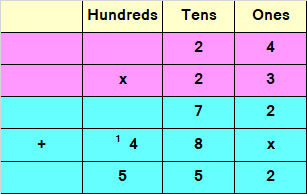# Multiplying 2 digit by 2 digit numbers

Multiplying 2 digit by 2 digit numbers – Question 1

Multiply :
23 x 31 = ?

Explanation

Step I: Whenever we multiply a two digit number by another 2 digit number, we first multiply the first number by ones place of second number, and then by first number by Tens place of the second number.
First we need to multiply 23 with 1
Multiply 1 by 3 in ones place.
1 x 3 = 3
Write 3 in ones place.
Multiply 1 by 2 in tens place.
1 x 2 = 2
Write 2 in tens place.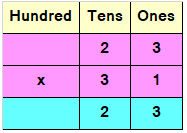Step II: Since the second number is a Tens value, we add a 0 or a cross to the answer obtained by multiplying first number by second digit
Multiply 23 with 3 and before multiplying write ‘x’ under 3
Multiply 3 by 3 in ones place.
3 x 3 = 9
Write 9 in tens place under 2
Multiply 3 by 2 in tens place.
3 x 2 = 6
Write 6 in hundreds place before 9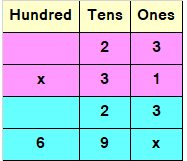Step III: Now add both the parts which have been multiplied.
3 + 0 = 3
2 + 9 = 11
Since, the number is more than 9, we need to carry over 1 to the hundreds place.
Add hundreds place and the carry of 1
6 + 1 = 7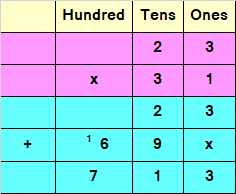Hence, 23 x 31 = 713

Multiplying 2 digit by 2 digit numbers – Question 2

Multiply :
24 x 23 = ?

Explanation

Step I: Whenever we multiply a two digit number by another 2 digit number, we first multiply the first number by
ones place of second number, and then by first number by Tens place of the second number.
First we need to multiply 24 with 3
Multiply 3 by 4 in ones place.
3 x 4 = 12
Since, the number is more than 9, we need to carry over 1 to the tens place.
Write 2 in ones place.
Multiply 3 by 2 in tens place.
3 x 2 = 6
Add the carry of 1 to 6 i.e, 1 + 6 = 7
Write 7 in tens place.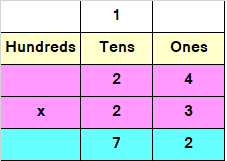Step II: Since the second number is a Tens value, we add a 0 or a cross to the answer obtained by multiplying first number by second digit
Multiply 24 with 2 and before multiplying write ‘x’ under 2
Multiply 2 by 4 in ones place.
2 x 4 = 8
Write 8 in tens place under 7
Multiply 2 by 2 in tens place.
2 x 2 = 4
Write 4 in hundreds place before 8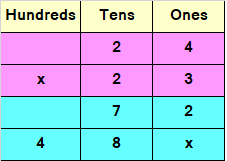Step III: Now add both the parts which have been multiplied.